The perfect place for easy learning...

×

# Python Tuple

In Python, a tuple is a collection of ordered and indexed elements of different data types. That means tuples are similar to the lists. But, the elements of a tuple are immutable however tuple itself is mutable. That means the elements of a tuple can not be modified whereas entire tuple can be modified or redefined. In Python, the tuple data type (data structure) has implemented with a class known as a tuple. All the elements of a tuple must be enclosed in parenthesis, and each element must be separated with a comma symbol. In Python, the tuple elements are organized as an array of elements of different data types. All the elements of a tuple are ordered and they are indexed. Here, the index starts from '0' (zero) and ends with 'number of elements - 1'.

• In Python, the tuple elements are also indexed with negative numbers from the last element to the first element in the tuple. Here, the negative index begins with -1 at the last element and decreased by one for each element from the last element to the first element.

## Creating a tuple in Python

The general syntax for creating a tuple in Python is as follows.

### Syntax

`tuple_name = (element_1, element_2, element_3, ...)`

For example, consider the following code for creating a tuple which stores the details of a student.

# Python code to illustrate creating a tuple

``````
student_data = (1, 'Rama', '2nd Year', 'CSE', 85.80)
print(type(student_data))
print(student_data)
```
```

When we run the above code, it produces the output as follows.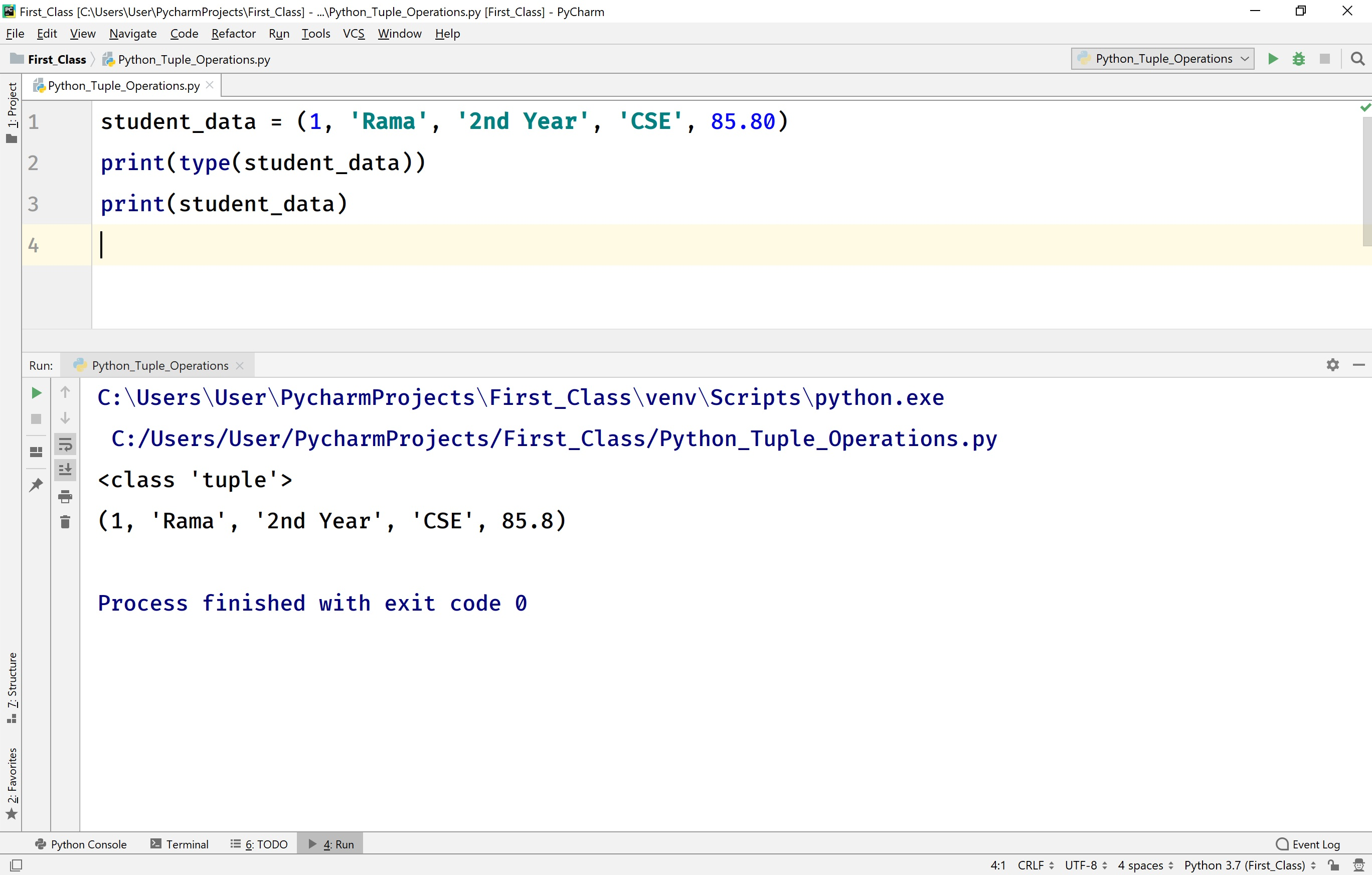In Python, a tuple can also be created using tuple( ) constructor. The tuple() constructor takes only one argument.

### Syntax

`tuple_name = tuple((element_1, element_2, element_3, ...))`

For example, consider the following code for creating a tuple using tuple() constructor which stores the details of a college.

# Python code to illustrate creating a tuple using tuple() constructor.

``````
college_info = tuple(('JNTUH', 'MRIT', 'MLTM'))
print(type(college_info))
print(college_info)
```
```

When we run the above code, it produces the output as follows.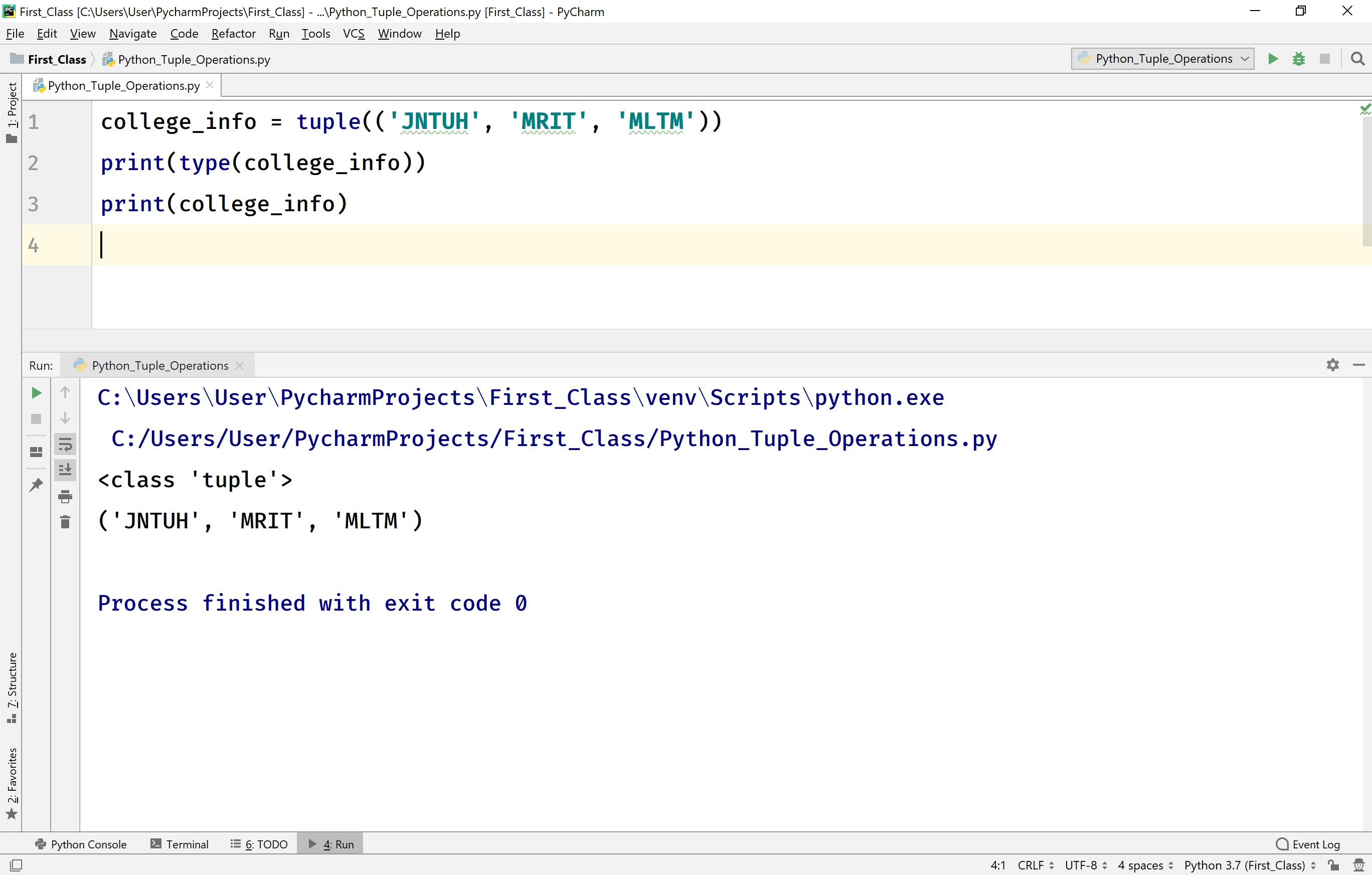## Accessing Elements of a tuple in Python

In Python, the tuple elements are organized using index values that start with '0' (zero) at first element and ends with 'length of the tuple - 1' at last element. The individual elements of a tuple are accessed using the index values.

### Syntax

`tuple_name[index]`

For example, consider the following code for accessing individual elements of a tuple.

# Python code to illustrate accessing elements of a tuple.

``````
student_data = (1, 'Rama', '2nd Year', 'CSE', 85.80)
print(type(student_data))
print(f'Roll Number: {student_data}\n'
f'Name of the Student: {student_data}\n'
f'Branch: {student_data}\n'
f'Year: {student_data}\n'
f'Percentage: {student_data}')
```
```

When we run the above code, it produces the output as follows.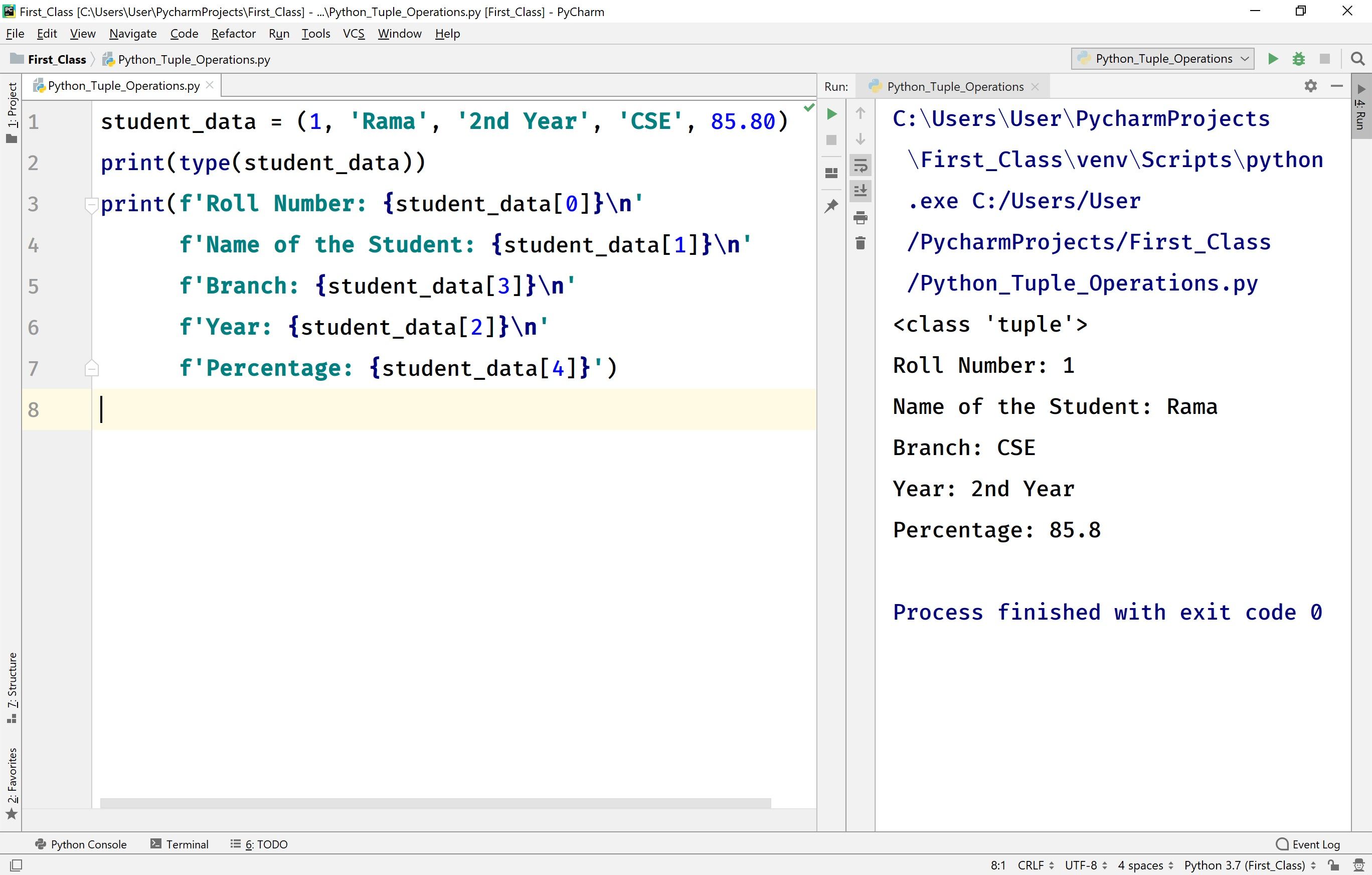In Python, we can also access a subset of elements from a tuple using slicing. We can access any subset of elements from the specified starting index to ending index. In tuple slicing, the default starting index is '0' and the default ending index is 'length of the tuple - 1'.

### Syntax

`tuple_name[starting_index : ending_index]`

For example, consider the following code for accessing a subset of elements from a tuple.

# Python code to illustrate accessing a subset of elements from a tuple.

``````
student_data = (1, 'Rama', '2nd Year', 'CSE', 85.80)
print(student_data[2:4]) # Accessing elements from index 2 to 3
print(student_data[:4]) # Accessing elements from index 0 to 3
print(student_data[2:]) # Accessing elements from index 2 to the last element
```
```

When we run the above code, it produces the output as follows.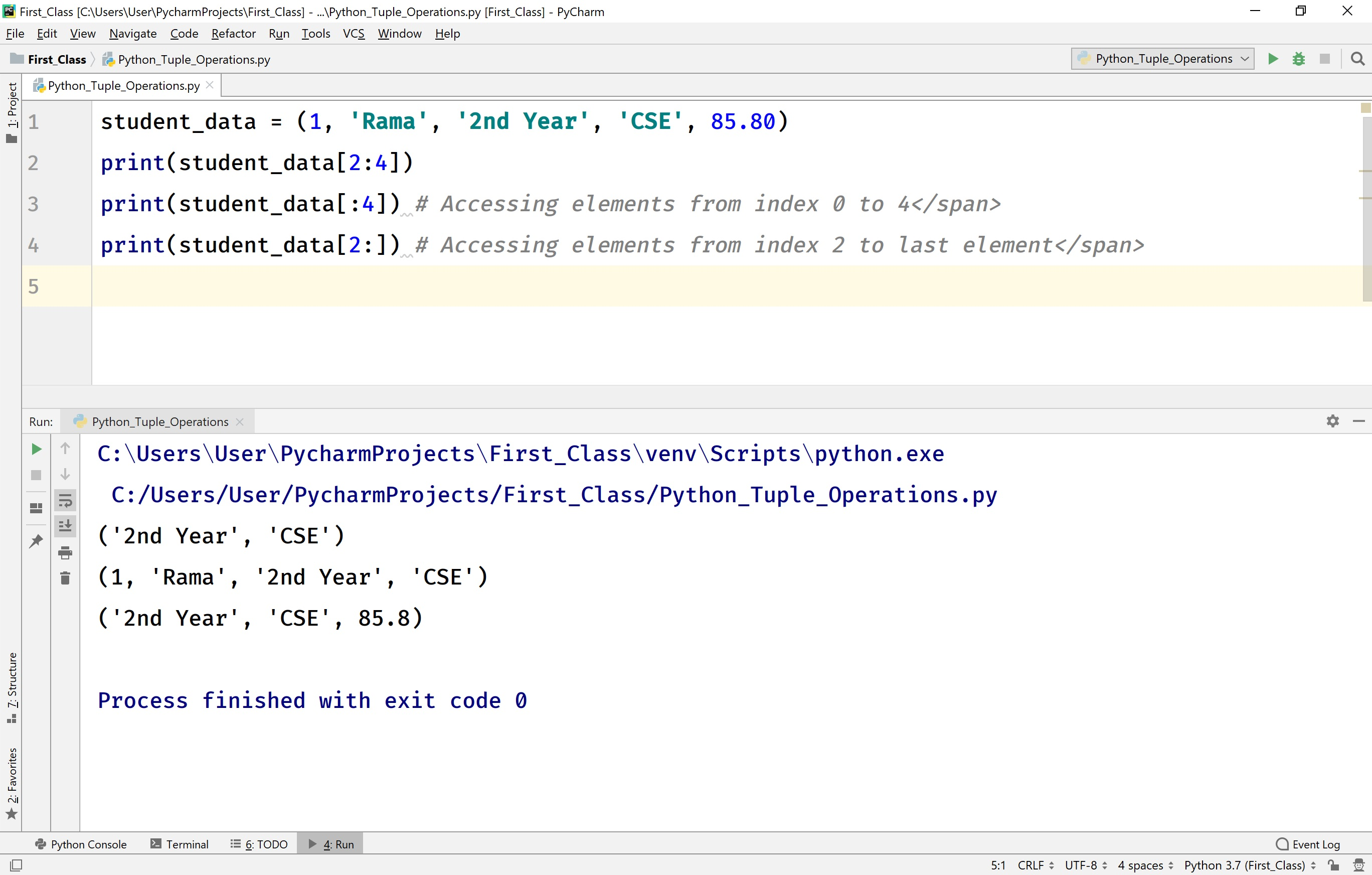## Changing an Element of a tuple in Python

In Python, the elements of a tuple are immutable. So, modification of individual elements in a tuple is not allowed.

• In Python, the whole tuple is mutable. So, we can modify or redefine the entire tuple.

## Looping through a tuple in Python

In Python, we can loop through a tuple using for statement with membership operator in.

For example, consider the following code to loop through a tuple.

# Python code to illustrate loop through a tuple.

``````
student_data = (1, 'Rama', '2nd Year', 'CSE', 85.80)
for element in student_data:
print(f'Element from the tuple is - {element}')
```
```

When we run the above code, it produces the output as follows.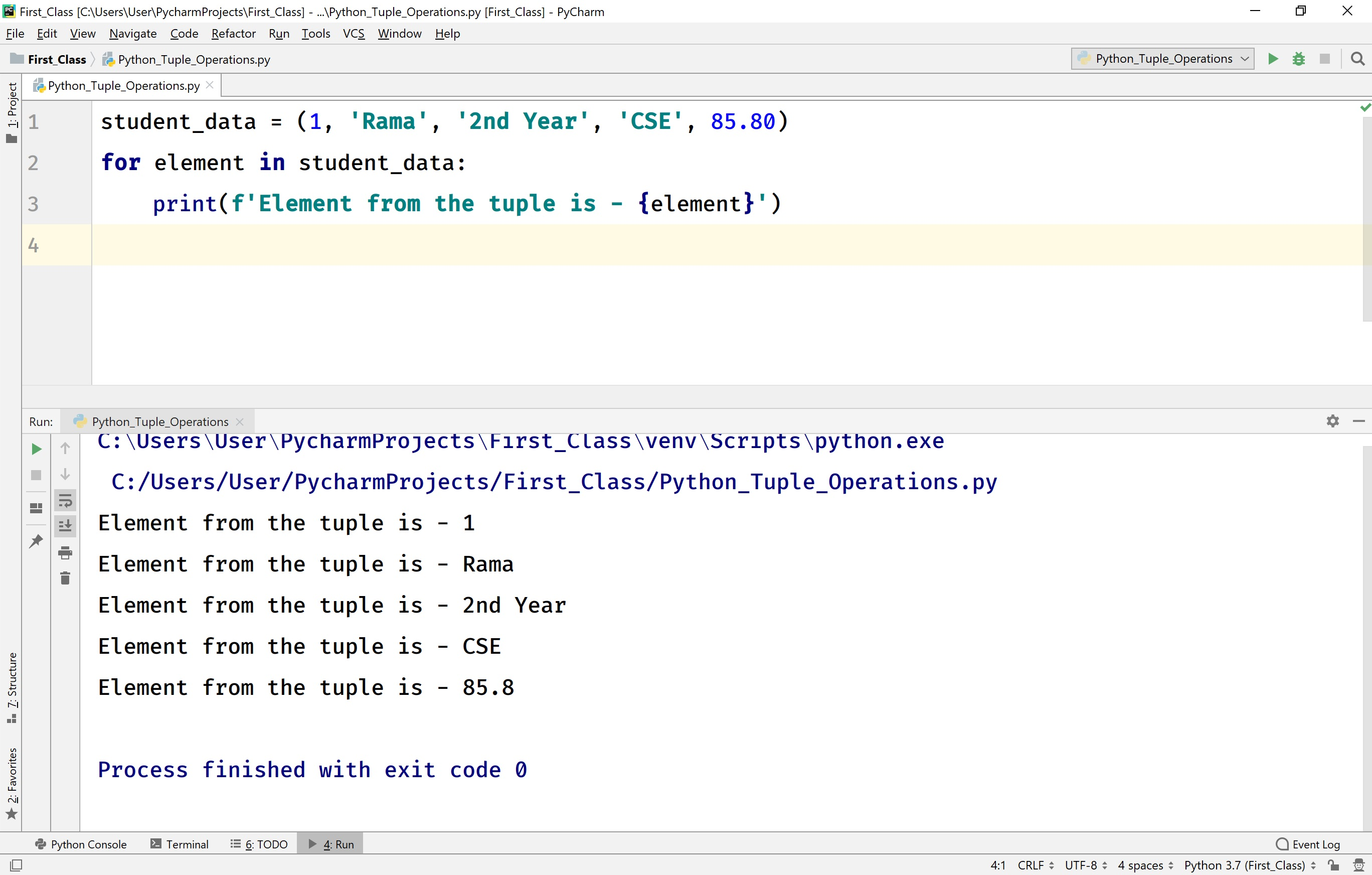## Existence of an element in a tuple in Python

In Python, we can test whether an element is present in a tuple or not using membership operator 'in'.

For example, consider the following code to test the existence of an element in a tuple.

# Python code to illustrate the existence of an element in a tuple.

``````
student_data = (1, 'Rama', '2nd Year', 'CSE', 85.80)
if 'CSE' in student_data:
print(f'CSE is found in the tuple {student_data}!!!')
else:
print(f'CSE is not found in the tuple {student_data}!!!')
```
```

When we run the above code, it produces the output as follows.## Finding the length of a tuple in Python

The Python provides a built-in function len( ) to find the length of a tuple. Here, the length of a tuple is the total number of elements in that tuple.

For example, consider the following code to find the length of a tuple.

# Python code to illustrate the length of a tuple.

``````
student_data = (1, 'Rama', '2nd Year', 'CSE', 85.80)
tuple_length = len(student_data)
print(f'Length of the tuple {student_data} is {tuple_length}')
```
```

When we run the above code, it produces the output as follows.## Adding an element to the tuple in Python

Adding an element to the existing tuple in Python is not allowed.

## Removing elements from a tuple in Python

In Python, removing an individual element from the existing tuple is not allowed. However, the entire tuple can be deleted using del keyword.

For example, consider the following code.

# Python code to illustrate del keyword using with a tuple.

``````
my_tuple = (1, 2, 3, 4, 5, 6, 7, 8, 9)
print(f'The tuple is - {my_tuple}')
del my_tuple
print(f'The tuple after del keyword is used - {my_tuple}') # GENERATES ERROR
```
```

When we run the above code, it produces the output as follows.## Counting the number of time a value appears in the tuple

The Python provides a built-in method called count(value) to count the number of times the given value appears in the tuple.

For example, consider the following code.

# Python code to illustrate the count( ) method in a tuple.

``````
my_tuple = (1, 2, 3, 4, 5, 6, 7, 8, 9, 2, 5, 2)
print(f'The tuple is - {my_tuple}')
print(f'The value 2 appears {my_tuple.count(2)} times in the tuple.')
```
```

When we run the above code, it produces the output as follows.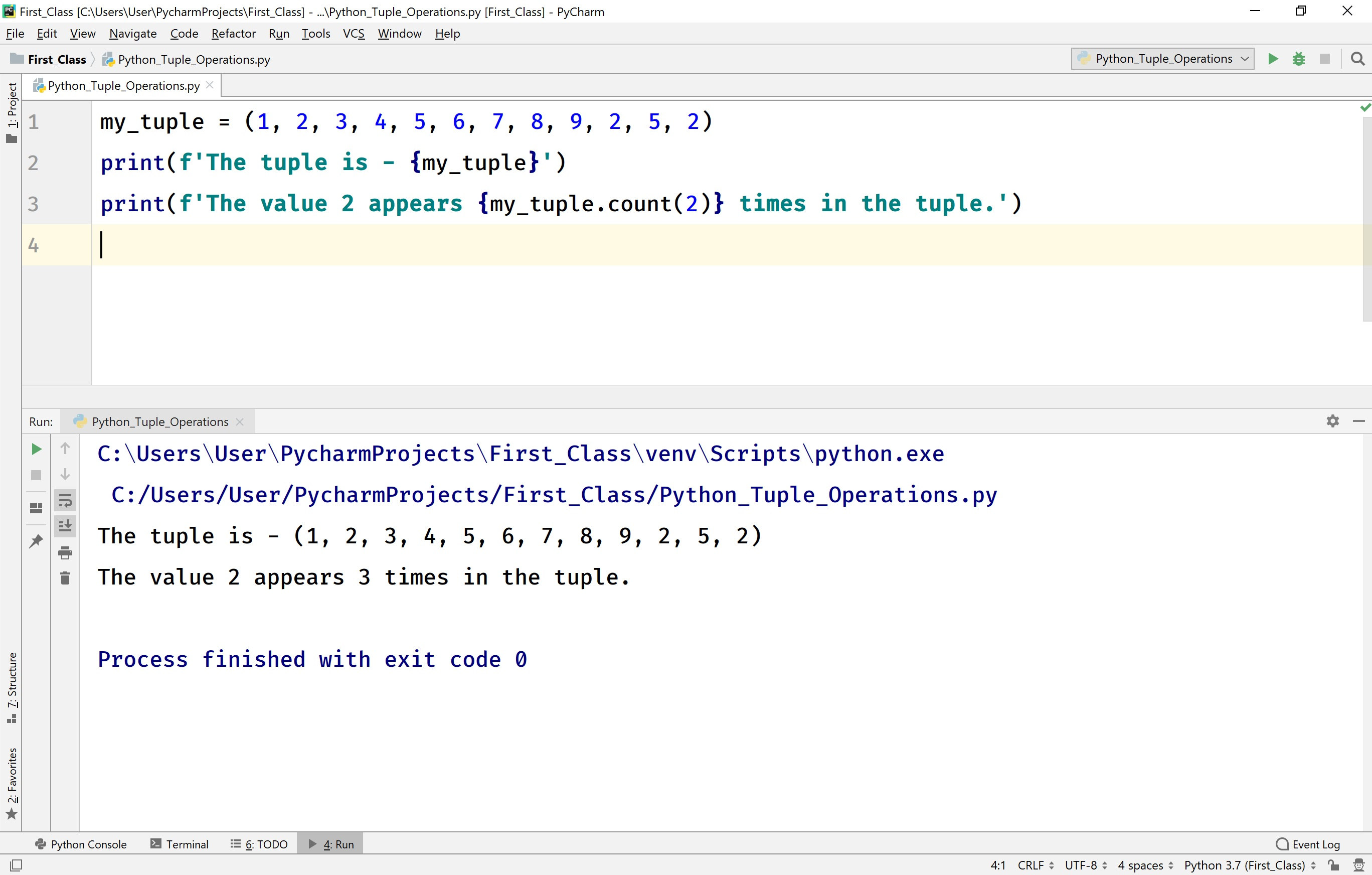## Finding the index of a value in a tuple

The Python provides a built-in method called index( value ) to find the index of that value in the tuple. If the given value not found in the tuple, then the execution terminates with an error message ValueError: <<value>> is not in a tuple. For example, consider the following example.

# Python code to illustrate the index( ) method in a tuple.

``````
my_tuple = (1, 2, 3, 4, 5)
print(f'The index of the value 4 is {my_tuple.index(4)}')
print(f'The index of the value 7 is {my_tuple.index(7)}') # generates an error
```
```

When we run the above code, it produces the output as follows.## Finding the Maximum and Minimum value in a tuple

The Python programming language provides built-in methods max( ) and min( ) to find the maximum and minimum elements in a tuple.

For example, consider the following code.

# Python code to illustrate the max( ) and mim( ) methods in a tuple.

``````
my_tuple = (12, 2, 5, 90, 30, 40, 3)
print(f'The maximum value in the tuple is - {max(my_tuple)}')
print(f'The minimum value in the tuple is - {min(my_tuple)}')
```
```

When we run the above code, it produces the output as follows.## Sorting the elements of a tuple in Python

The Python provides a built-in method sorted( ) to sort all the elements of a tuple. The method sorted( ) returns None. The sorted( ) method arranges the tuple elements in increasing order by default. To sort the elements in decreasing order, we need to pass an argument reverse = True to sorted( ) method.

For example, consider the following code.

# Python code to illustrate sort( ) in a tuple.

``````
my_tuple = (12, 2, 5, 90, 30, 40, 3)
print(f'The tuple is - {my_tuple}')
result_tuple = sorted(my_tuple)
print(f'Increasing order of the tuple is - {result_tuple}')
result_tuple = sorted(my_tuple, reverse=True)
print(f'Decreasing order of the tuple is - {result_tuple}')
```
```

When we run the above code, it produces the output as follows.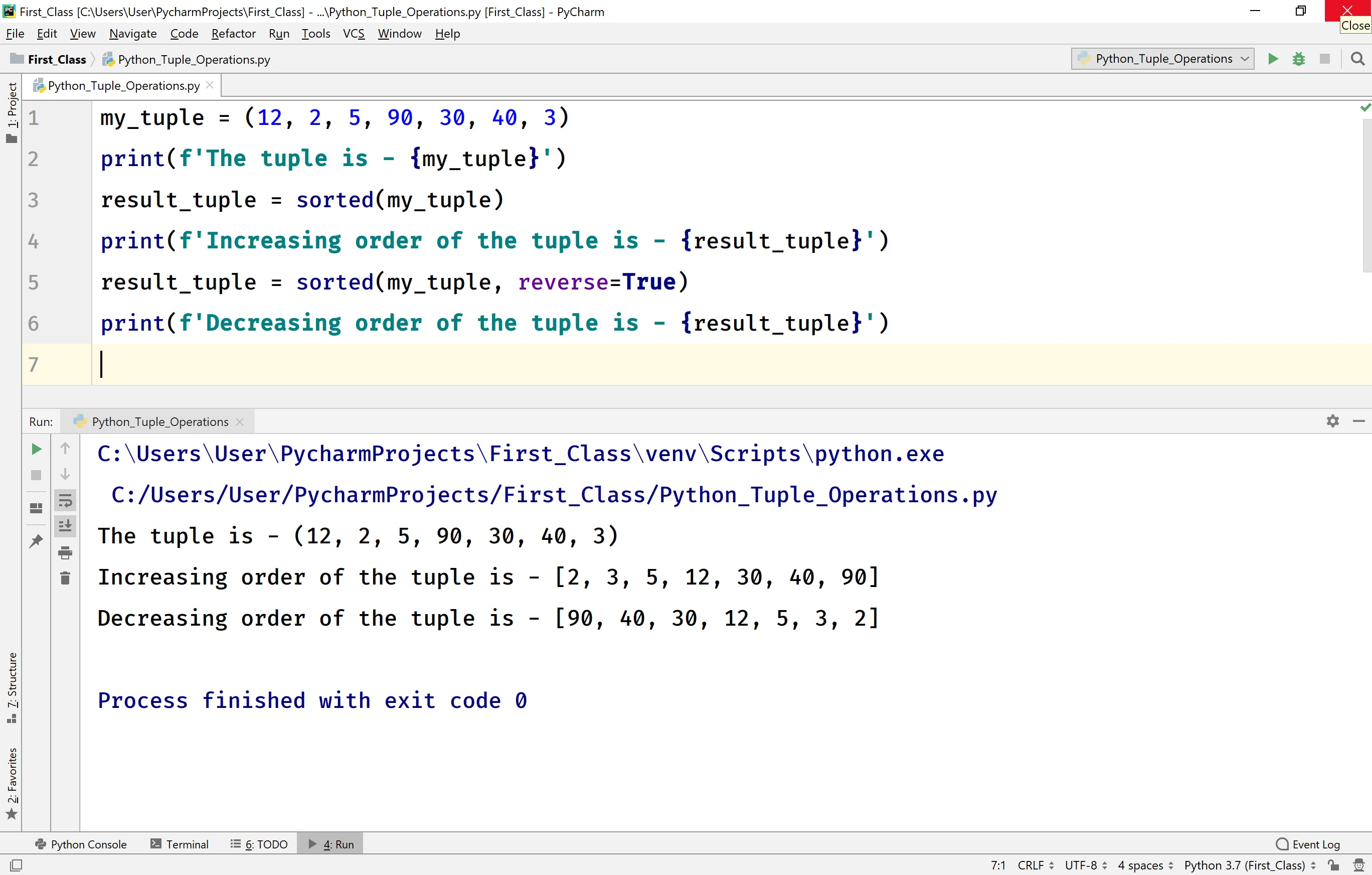• In Python, the functions max( ), min( ), and sorted( ) can be used with tuple only if the tuple contains all elements of numerical type.